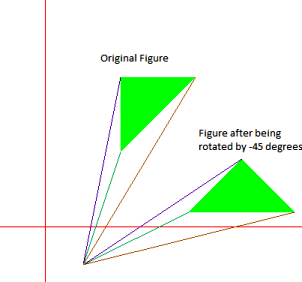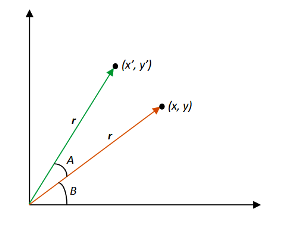# 2D Transformation | Rotation of objects

We have to rotate an object by a given angle about a given pivot point and print the new co-ordinates.

Examples:

Input : {(100, 100), (150, 200), (200, 200),
(200, 150)} is to be rotated about
(0, 0) by 90 degrees
Output : (-100, 100), (-200, 150), (-200, 200), (-150, 200)Input : {(100, 100), (100, 200), (200, 200)}
is to be rotated about (50, -50) by
-45 degrees
Output : (191.421, 20.7107), (262.132, 91.4214),
(332.843, 20.7107)## Recommended: Please try your approach on {IDE} first, before moving on to the solution.

In order to rotate an object we need to rotate each vertex of the figure individually.
On rotating a point P(x, y) by an angle A about the origin we get a point P'(x’, y’). The values of x’ and y’ can be calculated as follows:-We know that,
x = rcosB, y = rsinB

x’ = rcos(A+B) = r(cosAcosB – sinAsinB) = rcosBcosA – rsinBsinA = xcosA – ysinA
y’ = rsin(A+B) = r(sinAcosB + cosAsinB) = rcosBsinA + rsinBcosA = xsinA + ycosA

Rotational Matrix Equation:-## C

 // C program to rotate an object by   // a given angle about a given point  #include  #include     // Using macros to convert degree to radian  // and call sin() and cos() as these functions  // take input in radians  #define SIN(x) sin(x * 3.141592653589/180)  #define COS(x) cos(x * 3.141592653589/180)       // To rotate an object  void rotate(float a[], int n, int x_pivot,                         int y_pivot, int angle)  {      int i = 0;      while (i < n)      {          // Shifting the pivot point to the origin          // and the given points accordingly          int x_shifted = a[i] - x_pivot;          int y_shifted = a[i] - y_pivot;             // Calculating the rotated point co-ordinates          // and shifting it back          a[i] = x_pivot + (x_shifted*COS(angle)                             - y_shifted*SIN(angle));          a[i] = y_pivot + (x_shifted*SIN(angle)                             + y_shifted*COS(angle));          printf("(%f, %f) ", a[i], a[i]);          i++;      }  }     // Driver Code  int main()  {      // 1st Example      // The following figure is to be       // rotated about (0, 0) by 90 degrees      int size1 = 4;//No. of vertices         // Vertex co-ordinates must be in order      float points_list1[] = {{100, 100}, {150, 200},                                  {200, 200}, {200, 150}};           rotate(points_list1, size1, 0, 0, 90);             // 2nd Example      // The following figure is to be       // rotated about (50, -50) by -45 degrees      /*int size2 = 3;//No. of vertices      float points_list2[] = {{100, 100}, {100, 200},                                  {200, 200}};          rotate(points_list2, size2, 50, -50, -45);*/     return 0;  }

## CPP

 // C++ program to rotate an object by   // a given angle about a given point  #include  #include  using namespace std;     // Using macros to convert degree to radian  // and call sin() and cos() as these functions  // take input in radians  #define SIN(x) sin(x * 3.141592653589/180)  #define COS(x) cos(x * 3.141592653589/180)       // To rotate an object given as order set of points in a[]  // (x_pivot, y_pivot)  void rotate(float a[], int n, int x_pivot, int y_pivot,              int angle)  {      int i = 0;      while (i < n)      {          // Shifting the pivot point to the origin          // and the given points accordingly          int x_shifted = a[i] - x_pivot;          int y_shifted = a[i] - y_pivot;             // Calculating the rotated point co-ordinates          // and shifting it back          a[i] = x_pivot + (x_shifted*COS(angle)                             - y_shifted*SIN(angle));          a[i] = y_pivot + (x_shifted*SIN(angle)                             + y_shifted*COS(angle));          cout << "(" << a[i] << ", " << a[i] << ") ";          i++;      }  }     // Driver Code  int main()  {      // 1st Example      // The following figure is to be       // rotated about (0, 0) by 90 degrees      int size1 = 4;//No. of vertices      // Vertex co-ordinates must be in order      float points_list1[] = {{100, 100}, {150, 200},                                  {200, 200}, {200, 150}};           rotate(points_list1, size1, 0, 0, 90);             // 2nd Example      // The following figure is to be       // rotated about (50, -50) by -45 degrees      /*int size2 = 3;//No. of vertices      float points_list2[] = {{100, 100}, {100, 200},                                  {200, 200}};          rotate(points_list2, size2, 50, -50, -45);*/     return 0;  }

Output:

(-100, 100), (-200, 150), (-200, 200), (-150, 200)


References: Rotation matrix

This article is contributed by Nabaneet Roy. If you like GeeksforGeeks and would like to contribute, you can also write an article using contribute.geeksforgeeks.org or mail your article to contribute@geeksforgeeks.org. See your article appearing on the GeeksforGeeks main page and help other Geeks.

Attention reader! Don’t stop learning now. Get hold of all the important DSA concepts with the DSA Self Paced Course at a student-friendly price and become industry ready.

My Personal Notes arrow_drop_up

Improved By : Nexain

Article Tags :
Practice Tags :

3

Please write to us at contribute@geeksforgeeks.org to report any issue with the above content.